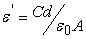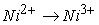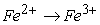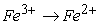﻿ Dielectric Studies of Single and Double Sintered Ni Substituted Li-Zn Ferrite

### Dielectric Studies of Single and Double Sintered Ni Substituted Li-Zn Ferrite

Ibetombi Soibam, Sanatombi SOPEN ACCESSPEER-REVIEWED

## Dielectric Studies of Single and Double Sintered Ni Substituted Li-Zn Ferrite

Ibetombi Soibam1,, Sanatombi S1

1Department of BS&H (Physics), NIT Manipur, Takyelpat, Imphal, India

### Abstract

Ni substituted Li-Zn ferrites with compositional formula Li0.4-0.5xZn0.2NixFe2.4-0.5xO4 where 0.02 ≤ x ≤ 0.1 in steps of 0.02 was synthesized by using citrate precursor method. X-ray diffraction was used to confirm the spinel phase. A series of sample was given sintering at 1080C and another series given sintering at 1080C after a pre-sintering at a temperature of 600C. The dielectric constant was investigated as a function of composition and frequency for the two series. Room temperature dielectric constant showed a decrease with addition of Ni2+ ions. It was explained in terms of space charge polarization and koops two layer model. For both the series the variation in dielectric constant with frequency showed dispersion. The possible mechanism responsible for the above behavior is being discussed.

### At a glance: Figures

12
Prev Next

• Soibam, Ibetombi, and Sanatombi S. "Dielectric Studies of Single and Double Sintered Ni Substituted Li-Zn Ferrite." American Journal of Materials Science and Engineering 2.3 (2014): 42-44.
• Soibam, I. , & S, S. (2014). Dielectric Studies of Single and Double Sintered Ni Substituted Li-Zn Ferrite. American Journal of Materials Science and Engineering, 2(3), 42-44.
• Soibam, Ibetombi, and Sanatombi S. "Dielectric Studies of Single and Double Sintered Ni Substituted Li-Zn Ferrite." American Journal of Materials Science and Engineering 2, no. 3 (2014): 42-44.

 Import into BibTeX Import into EndNote Import into RefMan Import into RefWorks

### 1. Introduction

Ferrites had been studied by many workers because of the varied technological application [1, 2]. They are found to be used at radio frequencies due to their characteristic dielectric properties. The dielectric properties of ferrites depend upon various factors like the synthesis method, type of substitutions and the sintering temperature [3, 4]. The study of composition and frequency dependence of dielectric behavior gives valuable information regarding localized charge carrier and dielectric polarization. Also it is generally accepted that lithium, probably as the oxide, volatilizes rapidly from lithium ferrite at high temperature if lithium is involved. In order to avoid this, synthesis of lithium ferrite at low temperature is preferred. Bismuth oxide had been generally used to lower down the sintering temperature. However search is going on for other methods too. It thus forms an important factor to investigate the sintering related phenomena. In the present work; the effect of single and double sintering on the dielectric properties of the citrate precursor prepared Ni substituted lithium ferrites is studied.

### 2. Experimental Details

Nickel substituted lithium ferrites with the formula Li0.4-0.5xZn0.2NixFe2.4-0.5xO4 was synthesized by the citrate precursor method. The starting precursors used were lithium nitrate, nickel nitrate, zinc nitrate, iron nitrate and citric acid. Stoichiometric amount of lithium nitrate, nickel nitrate, zinc nitrate, iron nitrate and citric acid were mixed to make a solution. The ratio of metal cations to citric acid is 1:1. The synthesis method had been reported earlier . The synthesized powder was mixed with a binder and pressed into pellets with 50 kilo pressure. The samples were pre-sintered at 600C followed by a final sintering in air at 1080C (DS). Another series of samples was given final sintering at 1080C without a pre sintering process (SS). From the XRD data the theoretical density was calculated for all the samples. Dielectric constant at room temperature was studied as a function of composition and frequency (20 Hz-1 MHz) for single and double sintered samples. The measurement was carried out using an Agilent E4980A Precision LCR meter and the value of dielectric constant was calculated using the formulawhere C is the measured capacitance, d the thickness, A the cross sectional area of the sample and ε0 the permittivity of free space.

### 3. Results and Discussion

3.1. X-ray Diffraction Patterns

XRD pattern was used to confirm the spinel phase structure of the Li0.4-0.5xZn0.2NixFe2.4-0.5xO4 ferrite sample. All the peaks could be indexed to the standard pattern reported by the Joint Committee on Powder Diffraction Standards (JCPDS). There is no extra peak observed showing that there is no impurity present. XRD for single sintered samples was reported earlier  whereas a typical XRD pattern for double sintered Li-Zn-Ni ferrite with concentration of Ni=0.02 is shown in Figure 1.

#### Table 1. Density (theoretical and experimental), Particle size of Li0.4-0.5xZn0.2NixFe2.4-0.5xO4Download asPowerPoint Slide

Veiw figureView current table in a new window

The densities of the samples calculated from the XRD data and those measured experimentally are tabulated in Table 1. An increase is observed with the increase in Ni ion concentration for both the series. These results are in agreement with the observations by previous workers [6, 7, 8]. The increase in densification may be due to the shrinking of the pores. Considering the single sintered and double sintered series of samples it is observed that densification is more in double sintered samples.

3.2. SEM Analysis

The particle size found out from SEM analysis shows an increasing trend with Ni ion concentration for both the series (Table 1). A typical micrograph for single sintered and double sintered samples of Ni concentration 0.02 is shown in Figure 2. Consequently double sintered samples show a larger particle size compared to single sintered samples. This is because the increase in density increases slowly the particle size.

3.3. Dielectric Properties

Figure 3 shows the variation of the room temperature dielectric constant (10 kHz) with Ni ion concentration. It is observed that the dielectric constant decreases with the increase in the Ni ion concentration for both the series. The decrease in the dielectric constant can be explained on the basis of space charge polarization and Koop’s two layer model. According to the model, ferrite is assumed to be made up of well conducting grains separated by grain boundaries. The electrical conduction in ferrite is explained by Verwey mechanism in terms of the hopping of electrons between Fe2+ and Fe3+ ions at B sites [9, 10]. The electrons reach the grain boundary by hopping and pile up due to its higher resistivity. This produces the space charge polarization. In the present study, the substitution with Ni2+ ions produces a change in the polarization so developed, as can be understood by considering the cationic formula,As Ni content is increased there is a decrease in Fe3+ ions and increase in Ni2+ ions at the B site. However, the exchange process ofis weak compared to, henceis assumed to be the dominant mechanism . The decrease in Fe3+ ion at the B site therefore decreases the hopping motion of electrons. This in turn decreases the piling up of electrons at the grain boundary, hence impeding the buildup of space charge polarization. The value of dielectric constant therefore decreases in both the series. However there is a decrease in the dielectric constant for double sintered samples. These may be due to decrease of charge mobility carrier with increasing sintering temperature. During oxidation in air at the time of sintering Fe2+ ion may be converted into Fe3+ ion suppressing the exchange of electron as was reported by other workers [11, 12]. Thus a decrease in the dielectric constant is observed with double sintering. But the phenomena need more supplementary investigations.

The frequency dependence of dielectric constant of single sintered samples is shown in Figure 4 and for double sintered samples in Figure 5. For both the series it is observed that the dielectric constant shows dispersion with increasing frequency which is a normal behavior of ferrites. At low frequencies the value of dielectric constant is high, and then decreases rapidly with increase in the frequency. The observed decrease in dielectric constant with frequency can be explained in terms of the space charge polarization and Koop’s two layer model as has been mentioned above . At low frequency of applied field the high resistivity grain boundary hinders the hopping motion of electrons creating space charge polarization, leading to a high dielectric constant. As the frequency of the applied field is increased the electronic exchange is not able to follow the alternating field and the electrons reverse the direction of motion thus decreasing the probability of electrons reaching the grain boundary. This leads to a decrease in the value of dielectric constant. At still higher frequency, the polarisability is very small and becomes independent of frequency.

### 4. Conclusion

Ni substituted lithium zinc ferrite had been prepared by citrate precursor method. The increase in the substitution of Ni concentration increases the density and the particle size but decreases the room temperature dielectric constant measured at 10 kHz. However the double sintered samples give more densification with an increase particle size. The dielectric constant at room temperature decreases with double sintering. Dispersion behavior is observed for the variation of dielectric constant with frequency. The dielectric constant is high at low frequency and decreases with the increase in the frequency for all the samples.

### Acknowledgement

The author would like to thank DST GoI (F.No.SR/FTP/PS-095/2012) for financial support.

### References

  I Soibam, S. Phanjoubam, C. Prakash, J. Magn. Magn. Mater., 321, 2779, (2009).In article CrossRef  J Smit, HPJ Wijn, Ferrites (Philips Technical Library) Eindhoven, Netherland, 1959.In article  A K Singh, TC Goel, RG Mendiratta, OP Thakur, C Prakash, J. Appl. Phys., 91, 6626, (2002).In article CrossRef  A K Singh, A Verma, O P Thakur, C Prakash, TC Goel, RG Mendiratta, Mater. Lett., 57, 1040, (2003).In article CrossRef  I Soibam, S Phanjoubam, C Prakash, J. Alloys Compd., 475, 328, 2009.In article CrossRef  I Soibam, S Phanjoubam, C Prakash, HC Verma, RK Kotnala, Mod. Phys. Lett. B, 24, 2277, (2010).In article CrossRef  N C Joshi, A Verma, N Kumar, O B S Kumar, S S Islam, Def. Sci. J., 57, 471, (2007).In article  R G Kharabe, R S Devan, C M Kanamadi, B K Chougule, Smart Mater. Struct., 15, N36, (2006).In article  EJW Verwey and JH De Boer, Recl. Trav. Chim. Pays. Bass, 55, 331, 1936.In article  EJW Verwey, PW Haayman and FC Romeijn J.Chem. Phys., 15, 181, 1947.In article CrossRef  CG Koops, Phys. Rev, 83, 121, (1951).In article CrossRef  A Tawfik, MM Barakat, J. mat. Sc. Lett., 7, 1098, (1988).In article CrossRef  I Soibam, S Phanjoubam, H B Sharma, H N K Sarma and C Prakash, Ind. J. Phys., 83, 285, 2009.In article CrossRef  AK Singh, TC Goel, RG Mendiratta, J.Appl. Phys., 91, 6626, 2002.In article CrossRef